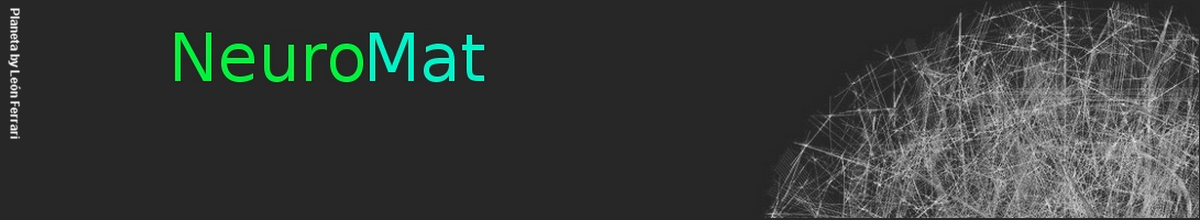### A Numerical Study of the Time of Extinction in a Class of Systems of Spiking Neurons

Cecilia Romaro, Fernando Araujo Najman, Morgan André

In this paper we present a numerical study of a mathematical model of spiking neurons introduced by Ferrari et al. in an article entitled Phase transition forinfinite systems of spiking neurons. In this model we have a countable number of neurons linked together in a network, each of them having a membrane potential taking value in the integers, and each of them spiking over time at a rate which depends on the membrane potential through some rate function ϕ. Beside being affected by a spike each neuron can also be affected by leaking. At each of these leak times, which occurs for a given neuron at a fixed rate γ, the membrane potential of the neuron concerned is spontaneously reset to 0.

A wide variety of versions of this model can be considered by choosing different graph structures for the network and different activation functions. It was rigorously shown that when the graph structure of the network is the one-dimensional lattice with a hard threshold for the activation function, this model presents a phase transition with respect to γ, and that it also presents a metastable behavior. By the latter we mean that in the sub-critical regime the re-normalized time of extinction converges to an exponential random variable of mean 1. It has also been proven that in the super-critical regime the renormalized time of extinction converges in probability to 1. Here, we investigate numerically a richer class of graph structures and activation functions. Namely we investigate the case of the two dimensional and the three dimensional lattices, as well as the case of a linear function and a sigmoid function for the activation function. We present numerical evidence that the result of metastability in the sub-critical regime holds for these graphs and activation functions as well as the convergence in probability to 1 in the super-critical regime.

The whole paper is available here.

NeuroCineMat
Featuring this week: